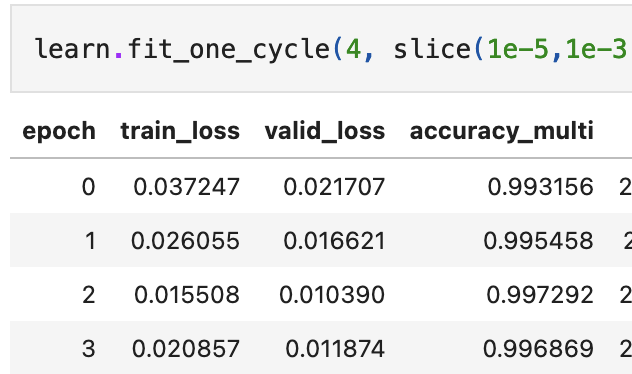# ConvNeXt in FastAI's Siamese Tutorial

Greetings,

Thank a lot Jeremy for the new 2022 course. I am trying to update some of my old models with content from the new course.

In the ConvNeXt Paper, the models appear to have a few differences over ResNet (in context of creating a custom Head for a Siamese Model):

1. Use of GELU and not ReLU
2. Removal of some Batch Norm layers
3. Substituting Batch Norm with Layer Norm

The current FastAI create_head() method utilizes ReLU and Batch Norm (example from docs):

``````Sequential(
)
(1): Flatten(full=False)
(2): BatchNorm1d(10, eps=1e-05, momentum=0.1, affine=True, track_running_stats=True)
(3): Dropout(p=0.25, inplace=False)
(4): Linear(in_features=10, out_features=512, bias=False)
(5): ReLU(inplace=True)
(6): BatchNorm1d(512, eps=1e-05, momentum=0.1, affine=True, track_running_stats=True)
(7): Dropout(p=0.5, inplace=False)
(8): Linear(in_features=512, out_features=10, bias=False)
)
``````

Questions:

1. Is this create_head() okay to use with a ConvNeXt based design for the Siamese Tutorial?
2. Any suggestions on “ConvNeXT-ifying” the current head for our Siamese Tutorial and use ConvNeXt instead of ResNet ?

Thanks and Warm Regards
-Gary

1 Like

Sounds interesting Gary – I haven’t tried it, so I suggest you give it a go and report back!Sure Thing.

Let me try to compare old runs with new training on a ConvNeXt body with a FastAI Head. Will report my findings.

Thanks and Warm Regards.
Gary

I ran the test in two parts, and compared it to the original Resnet implementation ( FasiAI’s Siamese Tutorial)

1. ConvNeXt Body with FastAi’s head
2. ConvNeXt Body with a new head that takes clues from the ConvNeXt paper, and has:
• No BatchNorm, has a single LayerNorm2D
• No Dropout (I just used the `Linear` portion of `LinBnDrop`)

Original Resnet Based Training(for reference) with these two parts. This old training was done using Resnet50:

Resnet `fit_one_cycle()` reference:

Resnet training/convergence reference:Part 1 : Test the same dataset, with ConvNeXt body, but with Resnet head from `create_head()`

The Last layers of a `convnext_t` model are as:

`````` CNBlock(
(block): Sequential(
(0): Conv2d(768, 768, kernel_size=(7, 7), stride=(1, 1), padding=(3, 3), groups=768)
(1): Permute()
(2): LayerNorm((768,), eps=1e-06, elementwise_affine=True)
(3): Linear(in_features=768, out_features=3072, bias=True)
(4): GELU(approximate=none)
(5): Linear(in_features=3072, out_features=768, bias=True)
(6): Permute()
)
(stochastic_depth): StochasticDepth(p=0.1, mode=row)
)
)
)
(classifier): Sequential(
(0): LayerNorm2d((768,), eps=1e-06, elementwise_affine=True)
(1): Flatten(start_dim=1, end_dim=-1)
(2): Linear(in_features=768, out_features=1000, bias=True)
)
``````

FasiAI’s `create_head()` chops off the last pooling layer, and adds this instead :

``````

Sequential(
)
(1): Flatten(full=False)
(2): BatchNorm1d(1536, eps=1e-05, momentum=0.1, affine=True, track_running_stats=True)
(3): Dropout(p=0.25, inplace=False)
(4): Linear(in_features=1536, out_features=1024, bias=False)
(5): ReLU(inplace=True)
(6): BatchNorm1d(1024, eps=1e-05, momentum=0.1, affine=True, track_running_stats=True)
(7): Dropout(p=0.5, inplace=False)
(8): Linear(in_features=1024, out_features=1, bias=False)
)

``````

Training with the same dataset:

`lr_find()` :

Training with `learn.freeze()`:

The model is not really able to utilize transfer learning from the ConvNeXt body.

Training with `learn.unfreeze()`

(Sorry, the kernel restarted here, but the model does’nt get much better than this and the error rate flattens around at around 95% accuracy)

Part 2 : Test the same dataset, with ConvNeXt body, but with ConvNeXt-based head (GELU, no BN, no Dropout etc)

``````Sequential(
)
(1): LayerNorm2d((1536,), eps=1e-06, elementwise_affine=True)
(2): Flatten(start_dim=1, end_dim=-1)
(3): Linear(in_features=1536, out_features=1024, bias=True)
(4): GELU(approximate=none)
(5): Linear(in_features=1024, out_features=1, bias=True)
)
``````

Output of last the few epochs :

This head too, taps-out at around 95% accuracy.

Results:

1. A Resnet based body appears to perform better than a ConNeXt body, no matter the choice of head. They had higher accuracy than both of the ConvNeXt experiments.
2. Resnets utilize transfer-learning much better than ConvNeXt. `learn.freeze()` training outputs give far better accuracy for Resnets.
3. Resnets converge really fast. ConvNeXts took much longer to converge. Maybe it is in line with this excerpt from ConvNeXt paper. ConvNeXts are trained for more than three-times the epochs for an equivalent Resnet.

Still trying to figure out how to improve ConvNeXt based training, over Resnets, for a Siamese NetworkWarm Regards
-Gary